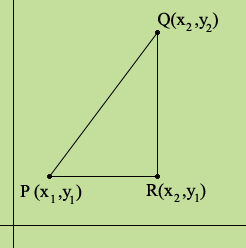SEARCH HOMEMath Central Quandaries & QueriesQuestion from supreet, a student: What are some real-world applications of the Pythagorean theorem? and How are the Pythagorean theorem and the distance formula related?Supreet,

For an answer to your first question go to the Quandaries and Queries page on Math Central and use the Quick Search to search for the term math beyond school pythagoras. You will see a number of questions we have received where our response uses the Pythagorean theorem.

For your second question place two points P and Q on the Cartesian plane and add a third point R so that triangle PQR is a right triangle with PQ the hypotenuse.Write the lengths of PR and RQ using the coordinates and then apply the Pythagorean theorem to this triangle.

HarleyMath Central is supported by the University of Regina and The Pacific Institute for the Mathematical Sciences.